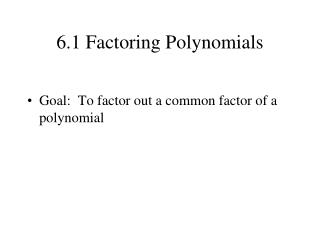DownloadDownload Presentation6.1 Factoring Polynomials

# 6.1 Factoring Polynomials

Download Presentation## 6.1 Factoring Polynomials

- - - - - - - - - - - - - - - - - - - - - - - - - - - E N D - - - - - - - - - - - - - - - - - - - - - - - - - - -
##### Presentation Transcript

1. 6.1 Factoring Polynomials • Goal: To factor out a common factor of a polynomial

2. Factoring (Divide): Convert a number or expression into an equivalent expression that is the product of two or more expressions. PRIME Factors

3. Relatively prime • No common factors Example: 4 and 7

4. Divisible by… 2 if last digit is an even number. 3 if the sum of the digits is divisible by 3 4 if the number formed by the last two digits is divisible by 4 5 if the number ends in 0 or 5 7 Nike Rule: “just do it” (long division) 9 if the sum of the digits  by 9 10 if the last digit is a zero.

5. Divisible by… 2 if last digit is an even number. 3 if the sum of the digits is divisible by 3 Is 97 divisible by 3? 97 is not divisible by 3

6. Divisible by… 2 if last digit is an even number. 3 if the sum of the digits is divisible by 3 Is 105 divisible by 3? 3 is a factor of 105

7. Divisible by… 2 if last digit is an even number. 3 if the sum of the digits is divisible by 3 Is 291 divisible by 3? 3 is a factor of 291

8. Divisible by… 2 if last digit is an even number. 3 if the sum of the digits is divisible by 3 4 if the number formed by the last two digits is divisible by 4 Is 291 divisible by 4? 4 is a not a factor of 291

9. Divisible by… 2 if last digit is an even number. 3 if the sum of the digits is divisible by 3 4 if the number formed by the last two digits is divisible by 4 Is 932 divisible by 4? 4 is a factor of 932

10. Is 9,365,126,210 divisible by 4? 4 is not a factor of 9,365,126,210

11. Is 3,228 divisible by 4? 4 is a factor of 3,228

12. “Factoring Polynomials”the REVERSE of the Distributive Property 5(x + 3) = 5x + 15

13. What # or variable is a factor of both terms? Factor: 10x + 15 2x + 3 Check your answer!!!!!

14. What # or variable is a factor of both terms? Factor: 12x + 4 3x + 1 Check your answer!!!!!

16. Factor x2 + 7x x•x + x •7 x(x + 7)

17. Factor a2b + 2ab a•a • b + 2 •a • b a(ab + 2b) ab(a + 2)

18. Factor 5x5y4 + 20xy3 5 x y3 ( ) x4y + 4 5xy3(x4y + 4)

19. Factor 16y5 + 20y3 4y3(4y2 + 5)

20. Factor x3(9 + 25x4)

21. Factor 3m3n(4m2n + 3m + 2n)

22. Factor 16x(x5 - 2x4 - 3)

23. Factor x2(6x2 - 10x + 3)

24. Factor 2a2(2a2b4 – ab2 + 6)

25. Which polynomial has 3x as a factor?

26. Homework Page 264 ( 10-50) even